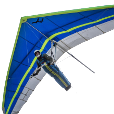# lISP ANGLE MEASUREMENT IN AUTOCAD 3D## Recommended PostsMy last try:

```(defun c:pp()
(setq p1 (cdr (assoc 10 (setq el (entget (car (entsel "Select line   \n "))))))
p2 (cdr (assoc 11 el))
ang1 (atan (distance p1 p3) (distance p2 p3))
ang2 (angle p3 p2)
)
(strcat  (convert ang1) "   |   " (convert ang2))
)
(defun convert(a)
(setq a (/ (* a 180.0) PI)
d (fix a)
m (fix (+ 0.5 (* 60.0 (- a d)))))
(strcat (itoa d) "deg " (itoa m) "min")
)```

• Replies 24
• Created

#### Top Posters In This Topic

•10

•7

•3

•2

#### Top Posters In This Topic

•CongVenh 10 posts

•fuccaro 7 posts

•lrm 3 posts

•BIGAL 2 posts

#### Posted Images14 hours ago, fuccaro said:

My last try:

```(defun c:pp()
(setq p1 (cdr (assoc 10 (setq el (entget (car (entsel "Select line   \n "))))))
p2 (cdr (assoc 11 el))
ang1 (atan (distance p1 p3) (distance p2 p3))
ang2 (angle p3 p2)
)
(strcat  (convert ang1) "   |   " (convert ang2))
)
(defun convert(a)
(setq a (/ (* a 180.0) PI)
d (fix a)
m (fix (+ 0.5 (* 60.0 (- a d)))))
(strcat (itoa d) "deg " (itoa m) "min")
)```

Thank you Fuccaro. The results were exactly what I wanted. Can you help me output that result to the screen instead of reading it from the command line?

##### Share on other sitesWhat do you mean by "output to the screen"? I could create two TEXT entities, but you will have to place them in the right position. You don't need any more to export the angles to Excel?

##### Share on other sites```(defun c:angles( / p1 p2 p3 ang1 ang2 sel a1 a2 textH)
(setq p1 (cdr (assoc 10 (setq el (entget (car (setq sel (entsel "Select line   \n ")))))))
p2 (cdr (assoc 11 el))
ang1 (atan (distance p1 p3) (distance p2 p3))
ang2 (angle p3 p2)
)
(strcat  (setq a1 (convert ang1)) "   |   " (setq a2 (convert ang2)))
(setq textH 2)
(entmake (mapcar 'cons '( 0 1 10 40 62) (list "text" a1 (cadr sel) textH 1)))
(entmake (mapcar 'cons '( 0 1 10 40 62) (list "text" a2 (mapcar '+ (cadr sel) (list textH textH 0)) textH 4)))
(export a1 a2)
)
(defun convert(a / d m)
(setq a (/ (* a 180.0) PI)
d (fix a)
m (fix (+ 0.5 (* 60.0 (- a d)))))
(strcat (itoa d) "deg " (itoa m) "min")
)
(defun export(a b / f file)
(setq file (getfiled "Specify out file" (substr (setq name (getvar "dwgname")) 1 (- (strlen name) 4))   "csv" 1))
(setq f (open file "w"))
(write-line a f)
(write-line b f)
(close f)
)```

•1
##### Share on other sites17 hours ago, fuccaro said:
```(defun c:angles( / p1 p2 p3 ang1 ang2 sel a1 a2 textH)
(setq p1 (cdr (assoc 10 (setq el (entget (car (setq sel (entsel "Select line   \n ")))))))
p2 (cdr (assoc 11 el))
ang1 (atan (distance p1 p3) (distance p2 p3))
ang2 (angle p3 p2)
)
(strcat  (setq a1 (convert ang1)) "   |   " (setq a2 (convert ang2)))
(setq textH 2)
(entmake (mapcar 'cons '( 0 1 10 40 62) (list "text" a1 (cadr sel) textH 1)))
(entmake (mapcar 'cons '( 0 1 10 40 62) (list "text" a2 (mapcar '+ (cadr sel) (list textH textH 0)) textH 4)))
(export a1 a2)
)
(defun convert(a / d m)
(setq a (/ (* a 180.0) PI)
d (fix a)
m (fix (+ 0.5 (* 60.0 (- a d)))))
(strcat (itoa d) "deg " (itoa m) "min")
)
(defun export(a b / f file)
(setq file (getfiled "Specify out file" (substr (setq name (getvar "dwgname")) 1 (- (strlen name) 4))   "csv" 1))
(setq f (open file "w"))
(write-line a f)
(write-line b f)
(close f)
)```

Thank you very much Fuccaro. Thanks to you, my work is easier

•1

## Join the conversation

You can post now and register later. If you have an account, sign in now to post with your account.
Note: Your post will require moderator approval before it will be visible.Unfortunately, your content contains terms that we do not allow. Please edit your content to remove the highlighted words below.

×   Pasted as rich text.   Restore formatting

Only 75 emoji are allowed.